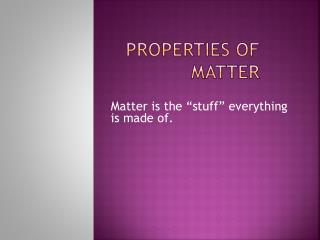Download PresentationProperties of Matter

# Properties of Matter - PowerPoint PPT PresentationDownload Presentation## Properties of Matter

- - - - - - - - - - - - - - - - - - - - - - - - - - - E N D - - - - - - - - - - - - - - - - - - - - - - - - - - -
##### Presentation Transcript

1. Properties of Matter Matter is the “stuff” everything is made of.

2. Inertia Things just don’t start moving or stop moving by themselves! RULES: The resistance of an object to changes in motion. 1. If an object is at rest, a force is needed to make it move. 2. If an object is moving, a force is needed to slow it.

3. Mass 1. The mass of an object is constant. Mass does not change as an object is moved from one place to another. For example, when you go to the moon, all your body parts are still attached even though you weigh less! The amount of matter in an object. 2. Measured in grams (g) or kilograms (kg).

4. Weight 1. All objects exert a gravitational attraction on other objects. A measure of the pull of gravity on an object. 2. For this reason, the weight of an object is not constant. (It depends on gravity.) 3. The SI unit of weight is the Newton (N).

5. volume 1. The SI unit for volume is the liter (L). The amount of space an object takes up. • Formula: • Length X Width X Height= cm³ • Formula (for an irregular shaped object): • End mL – Start mL= mL³

6. meniscus The point at the bottom of the curve of a liquid in a graduated cylinder.

7. How would you find the volume of this rock? 1. Immerse this rock in a graduated cylinder with water. (Be sure to note how much water was in the cylinder to start.) 2. Subtract the volume of the water alone from the volume of the water plus the rock. The answer is the volume of the rock.

8. density 1. How much stuff (matter) is in the space of the object (volume) is its density. or A specific property of matter that is related to its mass divided by the volume. (D=M/V) Which is more dense? In other words, which has more stuff crammed into the jar? One more thing: the density of a substance stays the same no matter how big the piece of the substance is. Water = 1 g/cm³ whether it’s in a glass or a lake.

9. Other measurements Time is measured in seconds (s). Temperature is commonly measured in degrees Celsius (C). It can also be measured using the Kelvin scale (K). This is the official SI unit because it uses no negative numbers. “0” is the coldest possible temperature.